Courses

# Semiconductor Physics

## 10 Questions MCQ Test Topicwise Question Bank for Electrical Engineering | Semiconductor Physics

Description
This mock test of Semiconductor Physics for Electrical Engineering (EE) helps you for every Electrical Engineering (EE) entrance exam. This contains 10 Multiple Choice Questions for Electrical Engineering (EE) Semiconductor Physics (mcq) to study with solutions a complete question bank. The solved questions answers in this Semiconductor Physics quiz give you a good mix of easy questions and tough questions. Electrical Engineering (EE) students definitely take this Semiconductor Physics exercise for a better result in the exam. You can find other Semiconductor Physics extra questions, long questions & short questions for Electrical Engineering (EE) on EduRev as well by searching above.
QUESTION: 1

### The mobility of free electrons and holes in pure germanium are 3800 and 1800 cm2/V-s respectively. The corresponding values for pure silicon are 1300 and 500 cm2/V-s, respectively. Assuming ni = 2.5 x 1013 cm-3 for germanium and ni = 1.5 x 1010 cm-3 for silicon at room temperature, the values of intrinsic conductivity for germanium and silicon are respectively given by

Solution:

The intrinsic conductivity for germanium is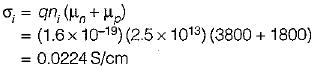The intrinsic conductivity for silicon is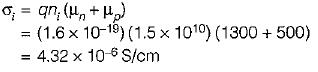QUESTION: 2

### What is the concentration of holes and electrons in n-type Silicon at 300°K, if the conductivity is 30 S/cm? Assume at 300°K, ni = 1.5 x 1010/cm3, μn = 1300 cm2/V-s and μp= 500 cm2/V-s

Solution:

The conductivity of an n-type silicon is a σ = qn μn.
Concentration of electrons,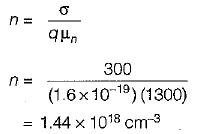Using “mass-action law'',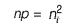∴ Concentration of holes,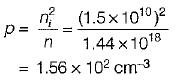QUESTION: 3

### When an electric field is applied across a semiconductor, free electrons in it will accelerate due to the applied field, and gain energy. This energy can be lost as heat when the electrons

Solution:

When an electron is accelerated by the potential applied to a semiconductor, the energy gained from the field may then be transferred to an atom when the electron collides with the atom.

QUESTION: 4

If a current of 1.6 μA is flowing through a conductor, the number of electrons crossing a particular cross-section per second will be

Solution:

Given, I = 1.6 x 10-6 A
= 1.6 x 10-6 Coulomb/second
Charge crossing a particular cross-section per second = 1.6 x 10-6 C Hence, number of electrons crossing a particular cross-section per second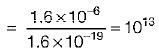QUESTION: 5

Match List-l with List-lI and select the correct answer using the codes given below the lists: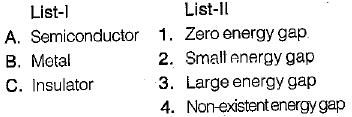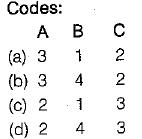Solution:

Energy band diagram for semiconductor, metal and insulator are shown below.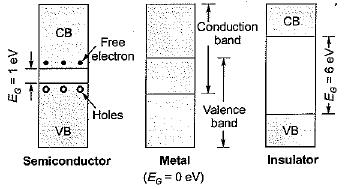QUESTION: 6

If elements in column IV of the periodic table are placed in increasing order of their atomic number, the order will be

Solution:
QUESTION: 7

Assertion (A): The drift velocity is in the direction opposite to that of the electric field.
Reason (R): At each inelastic collision with an ion, an electron loses energy, and a steady-state condition is reached where a finite value of drift speed is attained.

Solution:

Both assertion and reason are individually correct statements. However, the reason for assertion is that due to the applied electric field, and electrostatic force is developed on the electron and the electrons would be accelerated in a direction opposite to the applied electric field and this motion is called directed motion of electron.

QUESTION: 8

The density and mobility of electrons in a conductor are respectively 1020/cm3 and 800 cm2/V-s. If a uniform electric field of 1 V/cm exists across this conductor, then the electron current density would be approximately

Solution:

The current density is given by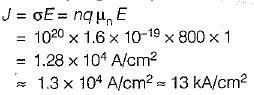QUESTION: 9

A semiconductor is doped with a donor density ND and no acceptors. If the intrinsic concentration is ni then the free electron density(n) will be equal to

Solution:

Given, NA = 0
Using “charge neutrality equation”, we have:
ND + P = NA + n
or, ND + p = ....(i)
Using'“Mass-action law”, we have: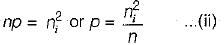Substituting value of p from equation (ii) in equation (i), we have: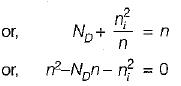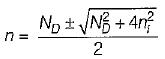Neglecting negative sign, we get: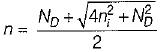= free electron density or concentration

QUESTION: 10

In a p-type semiconductor, p = 1016/cm2, and μp = 400 cm2/V-s. If a magnetic field (B) of 5 x 10-4 Weber/cm2 is applied in the x-direction, and an electric field of 2000 V/m is applied in +y direction. The value of electric field caused due to the “Hall effect” is

Solution:

The force acting on a charge q placed in a magnetic field B and an electric field E is given by
F = qv x B
The velocity of the hole placed in an electric field is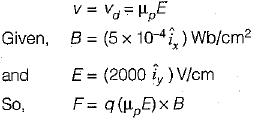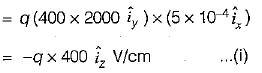Now, F - + q E ...(ii) (+q = charge on a hole)
Comparing equations (i) and (ii), the electric field due to the Hall effect will be -400 V/cm in +z direction.